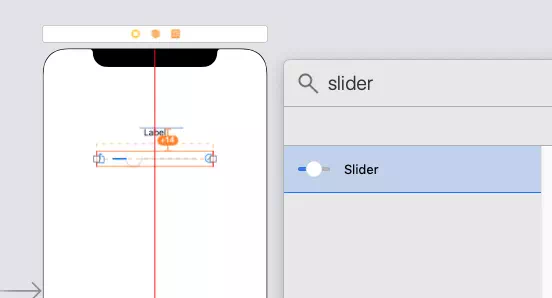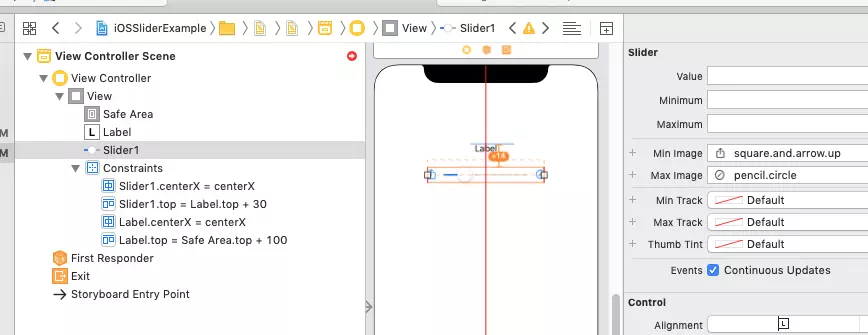# How To Customize Swift Slider In iOS App Example

This example will tell you how to use the swift UISlider class to create a slider in the iOS app. There are two sliders in this example. The above slider is added in the interface builder. The below slider is added in swift source code programmatically. When you slide the slider, the related slider’s value is displayed in the above label. Let us first look at the example below.

If you can not watch the above video, you can see it on URL https://youtu.be/qjjI3589s10

### 1. Add Swift Slider In Xcode Interface Builder.

1. Create an Xcode project using the Single View App template.
2. Click the Main.storyboard file to add a slider component to it.
3. Click Xcode menu item View —> Show Library to open Xcode UI component library window.
4. Input the keyword slider in the window search box, then drag and drop the slider component into the Main.storyboard screen. You should also add a label to the Main.storyboard file screen above the slider.5. You should add the below constraints to the label and slider object, and set the slider’s attributes ( value, minimum, maximum, Min Image, Max Image ) in the attributes inspector panel.### 2. Add Swift Slider And Set Its Properties In Swift Source Code.

Open the project’s ViewController.swift file. Then copy and paste below swift source code in it. The swift source code tells you the below functions.

1. how to add and config the iOS slider programmatically.
2. how to set ios slider’s minimum, maximum value, and image.
3. how to set ios slider’s background color.
4. how to change slider’s orientation.
5. how to make slider rounded corner.
```//
//  ViewController.swift
//  iOSSliderExample
//
//  Created by song zhao on 11/21/19.
//

import UIKit

class ViewController: UIViewController {

// The IBOutlet varibale refer to the label.
@IBOutlet weak var label: UILabel!
// The IBOutlet variable refer to slider1.
@IBOutlet weak var slider1: UISlider!

// slider2 variable is created in source code programmatically.
var slider2: UISlider = UISlider()

// Get iOS app device screen width and height.
let screenWidth:CGFloat = UIScreen.main.bounds.width
let screenHeight:CGFloat = UIScreen.main.bounds.height

initializeSlider1()

initializeSlider2()

}

/*
This function will initialize slider 1 object which is added in Main.storyboard file.
*/
func initializeSlider1(){

// Make slider1 width 0.8 times of screen width.
let widthConstraint = slider1.widthAnchor.constraint(equalTo: self.view.widthAnchor, multiplier: 0.8)
// Active above constraint.
widthConstraint.isActive = true

// Get slider1 value.
let slider1InitialValue:Float = slider1.value
// Cast above Float value to Int type value.
let slider1ValueInt:Int = Int(slider1InitialValue)

// Set slider1's value to the label text.
label.text = String(slider1ValueInt)

// Set slider1's background color.
slider1.backgroundColor = UIColor.green

// Set slider1's background corner radius to make the corner rounded.

}

/* This function will initilize the slider2 object which is added in source code programmatically. */
func initializeSlider2(){

// Set slider2's minimum and maxiun value.
slider2.minimumValue = 0
slider2.maximumValue = 300

// Get slider1's y location.
let slider1Y = slider1.frame.origin.y

// Get slider1's height.
let slider1Height = slider1.frame.height

// Create slider2's frame object of CGRect type.
let slider2Frame:CGRect = CGRect(x: 0, y: slider1Y + 300, width: screenWidth * 1, height: slider1Height)

// Set above CGRect object to slider2's frame attribute.
slider2.frame = slider2Frame

// Set slider2's background color.
slider2.backgroundColor = UIColor.yellow

// Set slider2's minimum value image, the image is a system image symbol. The system image name can be get from slider1's Attribute Inspector panel's Min Image or Max Image drop down list in Main.storyboard.
slider2.minimumValueImage = UIImage(systemName: "square.and.arrow.down")

// Set slider2's maximum value image, the image is a system image symbol.
slider2.maximumValueImage = UIImage(systemName: "square.and.arrow.up")

// Add slider2 to the iOS app main view.

// Change the slider2's direction from horizontal to vertical.
let trans:CGAffineTransform = CGAffineTransform(rotationAngle: CGFloat(Double.pi/2));
slider2.transform = trans;

// Add slider2's value changed process function.

}

// This function will be called when each slider's value is changed.
@IBAction func sliderValueChanged(_ sender: UISlider) {

// Get slider value Float type.
let sliderValue:Float = sender.value

// Cast Float type value to Int type.
let sliderValueInt:Int = Int(sliderValue)

// Set the Int type value to label text.
label.text = String(sliderValueInt)
}

}

```
Article Rating
Subscribe
Notify ofThis site uses Akismet to reduce spam. Learn how your comment data is processed.